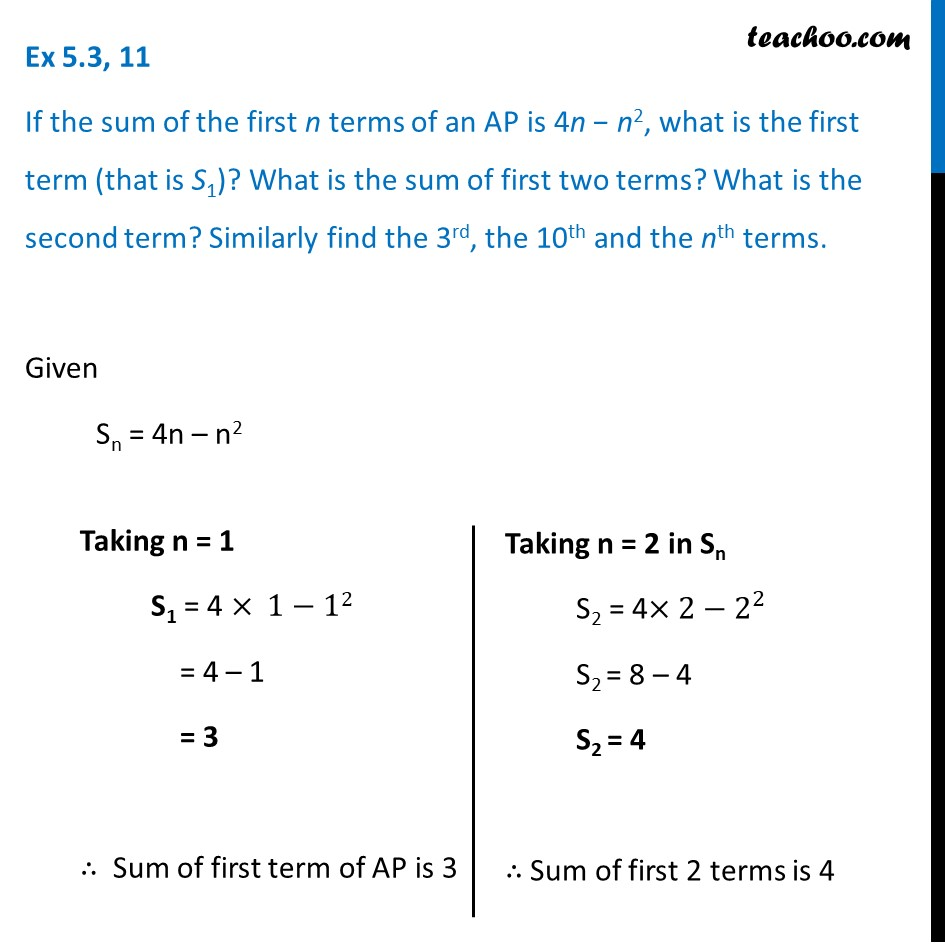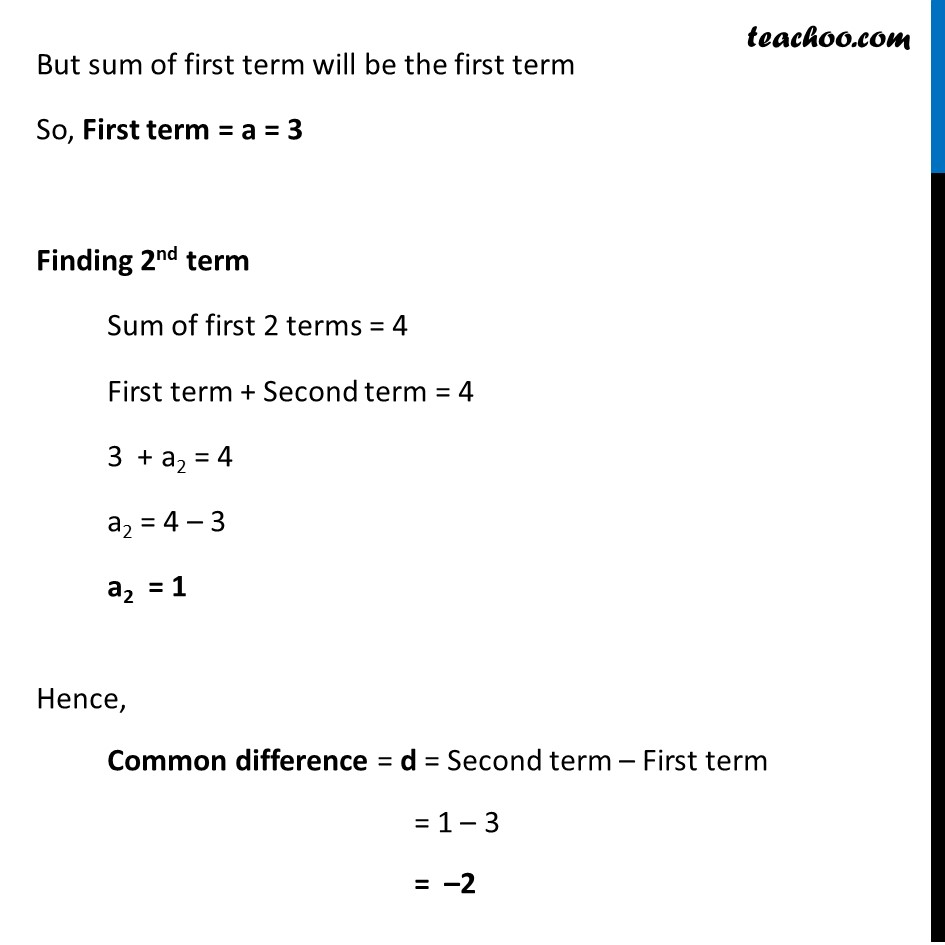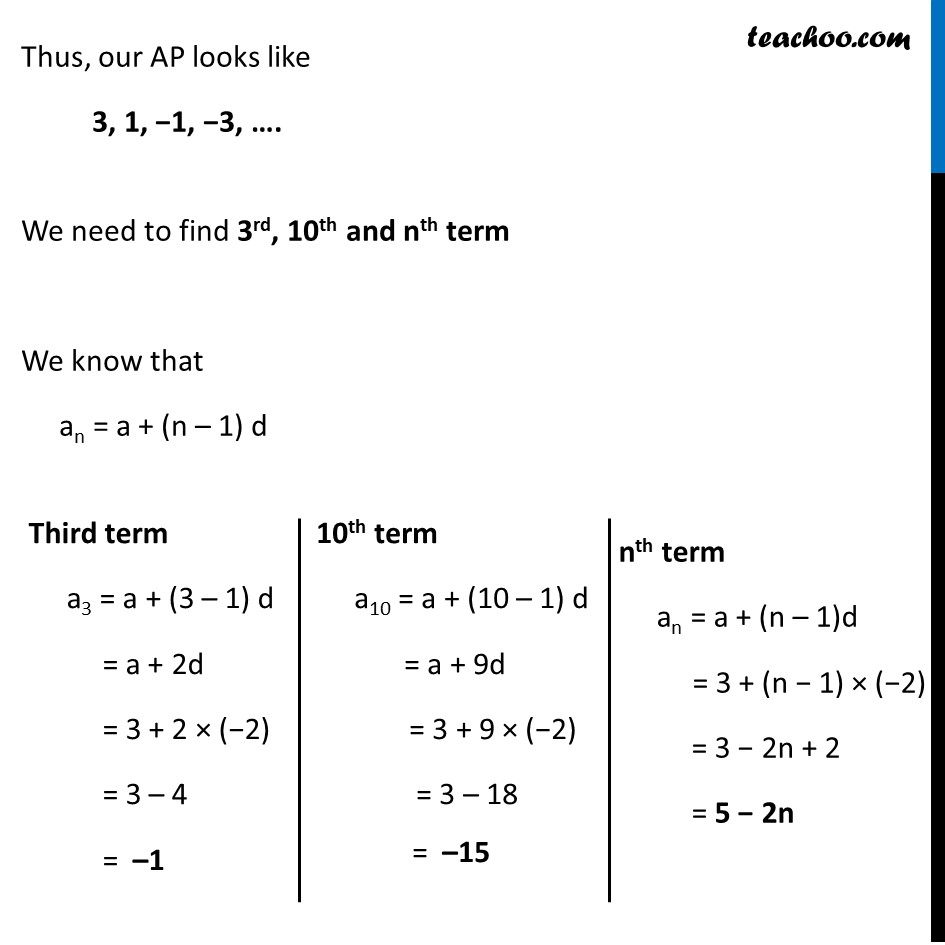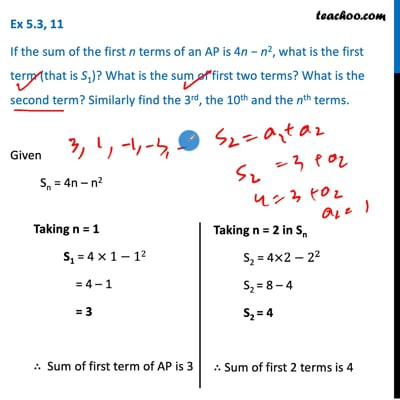Ex 5.3

Chapter 5 Class 10 Arithmetic Progressions (Term 2)
Serial order wiseThis video is only available for Teachoo black users

### Transcript

Ex 5.3, 11 If the sum of the first n terms of an AP is 4n − n2, what is the first term (that is S1)? What is the sum of first two terms? What is the second term? Similarly find the 3rd, the 10th and the nth terms. Given Sn = 4n – n2 Taking n = 1 S1 = 4 × 1−12 = 4 – 1 = 3 ∴ Sum of first term of AP is 3 Taking n = 2 in Sn S2 = 4×2−2^2 S2 = 8 – 4 S2 = 4 ∴ Sum of first 2 terms is 4 But sum of first term will be the first term So, First term = a = 3 Finding 2nd term Sum of first 2 terms = 4 First term + Second term = 4 3 + a2 = 4 a2 = 4 – 3 a2 = 1 Hence, Common difference = d = Second term – First term = 1 – 3 = –2 Thus, our AP looks like 3, 1, −1, −3, …. We need to find 3rd, 10th and nth term We know that an = a + (n – 1) d Third term a3 = a + (3 – 1) d = a + 2d = 3 + 2 × (−2) = 3 – 4 = –1 10th term a10 = a + (10 – 1) d = a + 9d = 3 + 9 × (−2) = 3 – 18 = –15 nth term an = a + (n – 1)d = 3 + (n − 1) × (−2) = 3 − 2n + 2 = 5 − 2n